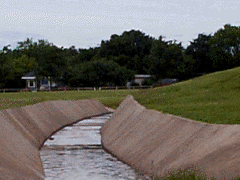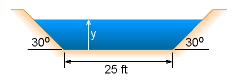Ch 10. Open-Channel Flow Multimedia Engineering Fluids UniformFlow GraduallyVaried Flow Rapidly VariedFlow
 Chapter 1. Basics 2. Fluid Statics 3. Kinematics 4. Laws (Integral) 5. Laws (Diff.) 6. Modeling/Similitude 7. Inviscid 8. Viscous 9. External Flow 10. Open-Channel Appendix Basic Math Units Basic Equations Water/Air Tables Sections Search eBooks Dynamics Fluids Math Mechanics Statics Thermodynamics Author(s): Chean Chin Ngo Kurt Gramoll ©Kurt GramollFLUID MECHANICS - CASE STUDY IntroductionWater Channel A city is considering building a water channel which ends in a free waterfall in a park. A hydraulic engineer is asked to determine the water surface profile for a discharge flow rate of 750 ft3/s. The channel has a trapezoidal shape with a base width of 25 ft, and the angles on both sides are 30o as shown in the figure. The slope of the channel is 0.0015. Questions Determine the critical and normal depths, and identify the surface profile type. Then compute the water surface profile using the direct step method. ApproachDimensions of the Channel Assume the flow is gradually varied flow. Assume the Manning coefficient is 0.025 for gravelly excavated earth channel*. * For practices, students are encouraged to repeat this problem using the Manning coefficient for concrete (shown in the picture).

Practice Homework and Test problems now available in the 'Eng Fluids' mobile app
Includes over 250 problems with complete detailed solutions.
Available now at the Google Play Store and Apple App Store.